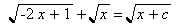MAT103
Franklin College
Erich Prisner

# A family of radical equationsLook at the following family of radical equations (with parameter c, for each number c we get another equation). Solve this equation for c = 1/2. Solve it also for c=-2. Then find out for which values of c there is a solution.

Erich Prisner, August 2003